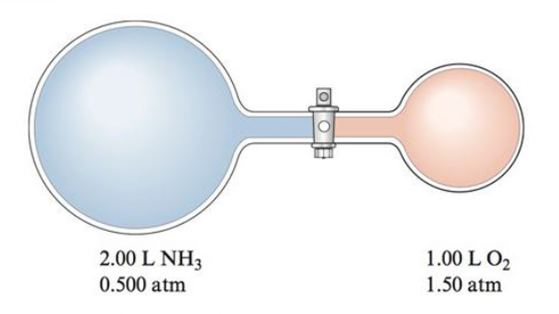# Nitric acid is produced commercially by the Ostwald process. In the first step ammonia is oxidized to nitric oxide: 4NH 3 ( g ) + 5O 2 ( g ) → 4NO ( g ) + 6H 2 O ( g ) Assume this reaction is carried out in the apparatus diagramed below. The stopcock between the two reaction containers is opened, and the reaction proceeds using proper catalysts. Calculate the partial pressure of NO after the reaction is complete. Assume 100% yield for the reaction, assume the final container volume is 3.00 L, and assume the temperature is constant.### Chemistry: An Atoms First Approach

2nd Edition
Steven S. Zumdahl + 1 other
Publisher: Cengage Learning
ISBN: 9781305079243

#### Solutions

Chapter
Section### Chemistry: An Atoms First Approach

2nd Edition
Steven S. Zumdahl + 1 other
Publisher: Cengage Learning
ISBN: 9781305079243
Chapter 8, Problem 136AE
Textbook Problem
2191 views

## Nitric acid is produced commercially by the Ostwald process. In the first step ammonia is oxidized to nitric oxide: 4NH 3 ( g )   +  5O 2 ( g )   →  4NO ( g )   +  6H 2 O ( g ) Assume this reaction is carried out in the apparatus diagramed below.The stopcock between the two reaction containers is opened, and the reaction proceeds using proper catalysts. Calculate the partial pressure of NO after the reaction is complete. Assume 100% yield for the reaction, assume the final container volume is 3.00 L, and assume the temperature is constant.

Interpretation Introduction

Interpretation: The partial pressure of NO in the given reaction is needed to be determined.

Concept introduction:

• According to Boyle’s law of gas molecules,

If the volume of gases in a container is increased then the pressure will decrease at constant temperature and number of moles.

Equation for Boyle’s law is,

PinitialVinitial=PfinalVfinal

• Mole ratios between the reactant and products of a reaction are depends upon the coefficients of respective reactant in a balanced chemical equation.
• Limiting reagent: limiting reagent is a reactant, which consumes completely in the chemical reaction. The quantity of the product depends on this limiting reagent, it can be determined with the help of balanced chemical equation for the reaction.
• According toideal gas law: the pressure of gas is directly proportional to the number of moles of gas at constant volume and temperature.

Pn=RTV , P and n are proportional.

Mathematically, for two gases (1 and 2) at constant V and T,

P1n1=P2n2P1P2=n1n2

### Explanation of Solution

Explanation

• To find: the final partial pressures of NH3 and O2 gas in the given figure.

Final partial pressure of NH3 in the figure is 0.333atm .

Final partial pressure of O2 in the figure is 0.500atm .

• In the figure,

The initial pressure of NH3 gas is 0.500atm

The initial volume of NH3 gas is 2L

The final volume is given as 3L

Equation for Boyle’s law is,

PinitialVinitial=PfinalVfinal

Therefore,

Final partial pressure of NH3 is,

Pfinal=0.500atm×2L3L =0.333atm

• In the figure,

The initial pressure of O2 gas is 1.5atm

The initial volume of O2 gas is 1L

The final volume is given as 3L

Equation for Boyle’s law is,

PinitialVinitial=PfinalVfinal

Therefore,

Final partial pressure of O2 is,

Pfinal=1.5atm×1L3L =0.500atm

• To find: the molar ratios of produced NO to the reactants NH3 and O2 in the given reaction.

The mole ratio between NO and NH3 is 4:4 .

The mole ratio between NO and O2 is 4:5 .

The balanced equation of the given reaction is 4NH3+5O24NO+6H2O .

Here the 4 moles of NO is produced by 4 moles of NH3 and 5 moles of O2 .

Therefore,

The mole ratio between NO and NH3 is,

4:4

The mole ratio between NO and O2 is,

4:5

• To find: the limiting reagent in the given reaction.

The limiting reagent in the given reaction is NH3 .

The final partial pressure of NH3 in the figure is 0.333atm .

The final partial pressure of O2 in the figure is 0.500atm .

The mole ratio between NO and NH3 is 4:4 .

The mole ratio between NO and O2 is 4:5 .

The balanced equation of given reaction is,

4NH3+5O24NO+6H2O

The reaction is carried under constant volume and temperature.

According to ideal gas law, the pressure of gas is directly proportional to the number of moles of gas at constant volume and temperature.

Pn=RTV , P and n are proportional.

Mathematically, for two gases (1 and 2) at constant V and T,

P1n1=P2n2P1P2=n1n2

Therefore,

• The relationship between NO and NH3 is,

PNO0

### Still sussing out bartleby?

Check out a sample textbook solution.

See a sample solution

#### The Solution to Your Study Problems

Bartleby provides explanations to thousands of textbook problems written by our experts, many with advanced degrees!

Get Started

Find more solutions based on key concepts
The roles of protein in the body include all but a. blood clot formation. b. tissue repair. c. gas exchange. d....

Nutrition: Concepts and Controversies - Standalone book (MindTap Course List)

What are the moral implications of using ergogenic aids?

Understanding Nutrition (MindTap Course List)

What makes up a system of units?

An Introduction to Physical Science

Why does cyclohexane assume a chair form rather than a planar hexagon?

Chemistry for Today: General, Organic, and Biochemistry

What are some functions of skin?

Human Biology (MindTap Course List)

Does the cell cycle refer to mitosis as well as meiosis?

Human Heredity: Principles and Issues (MindTap Course List)

(a) Evaluate A in the scalar equality 4 (7 + 3) = A. (b) Evaluate A, B, and C in the vector equality 700 + 3.00...

Physics for Scientists and Engineers, Technology Update (No access codes included)